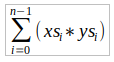# Problem Sheet for LI Functional Programming - Week 3

## List Comprehensions​

1. A triple (x,y,z) of positive integers is called pythagorean if $x^2 + y^2 = z^2$. Using a list comprehension, define a function:

hspyths :: Int -> [(Int,Int,Int)]that maps an integer n to all such triples with components in [1..n]. For example:hs> pyths 5[(3,4,5),(4,3,5)]
2. A positive integer is perfect if it equals the sum of all of its factors, excluding the number itself. Using a list comprehension, define a function

perfects :: Int -> [Int]

that returns the list of all perfect numbers up to a given limit. For example:

> perfects 500[6,28,496]

Many variations of this exercise are possible:

• A number which is less than the sum of its proper divisors is called abundant.
• A number which is greater than the sum of its proper divisions is called deficient.
• A number for which the sum of all its divisors (including itself) is greater than the sum of the divisors of any smaller number is called highly abundant.

For each of these variations, write a function which finds all the numbers with the stated property below a given number.

3. The scalar product of two lists of integers xs and ys of length n is give by the sum of the products of the corresponding integers:Using a list comprehension, define a function that returns the scalar product of two lists.

4. Harder Implement matrix multiplication where matricies are represented by lists of lists of integers. One possibility, for example, would be to take the dimensions of the matrices as arguments, so that your function would have type:

  martrix_mul :: Int -> Int -> [[Int]] -> [[Int]] -> [[Int]]

## 递归函数 Recursive Functions​

1. Without looking at the standard prelude, define the following library functions using recursion:

• Decide if all logical values in a list are true:

and :: [Bool] -> Bool
• Concatenate a list of lists:

concat :: [[a]] -> [a]
• Produce a list with n identical elements:

replicate :: Int -> a -> [a]
• Select the nth element of a list:

(!!) :: [a] -> Int -> a
• Decide if a value is an element of a list:

elem :: Eq a => a -> [a] -> Bool
2. Define a recursive function

merge :: Ord a => [a] -> [a] -> [a]

that merges two sorted lists of values to give a single sorted list.

For example:

> merge [2,5,6] [1,3,4][1,2,3,4,5,6]

## 高阶函数 Higher Order Functions​

1. What are higher-order functions that return functions as results better known as?

2. Express the comprehension [f x | x <- xs, p x] using the functions map and filter. The function type is given as:

fun :: Num a => (a -> a) -> (a -> Bool) -> [a] -> [a]

For example:

> fun (^2) even [1..20][4,16,36,64,100,144,196,256,324,400]> fun (^2) odd [1..20][1,9,25,49,81,121,169,225,289,361]
3. Redefine map f and filter p using foldr. For your reference, here are the definitions of map and filter from lecture notes.

map :: (a -> b) -> [a] -> [b]map f []     = []map f (x:xs) = f x : map f xsfilter :: (a -> Bool) -> [a] -> [a]filter p [] = []filter p (x:xs)   | p x       = x : filter p xs   | otherwise = filter p xs
4. Define a function altMap :: (a -> b) -> (a -> b) -> [a] -> [b] that alternatively applies the two argument functions to successive elements in a list.

For example:

> altMap (+10) (+100) [0,1,2,3,4][10,101,12,103,14]
5. Harder. Church Numerals

It is possible to represent the natural numbers (i.e. 0,1,2,...) using higher order functions of type

(a -> a) -> (a -> a)

These are called Church Numerals. The encoding works like this: the input to a function of the above type is an element f of type a -> a. Since such a function takes input values of type a and also produces output values of type a, this means it can be iterated. So we will represent the numeral n by a the element of type (a -> a) -> (a -> a) which iterates its argument n times.

To see the idea, the first few examples of numerals are written like this:

zero :: (a -> a) -> (a -> a)zero f x = xone :: (a -> a) -> (a -> a)one f x = f xtwo :: (a -> a) -> (a -> a)two f x = f (f x)three :: (a -> a) -> (a -> a)three f x = f (f (f x))
• Write a function to implement addition of Church numerals
• Write a function to implement multiplication of Church numerals